# Resources tagged with: Place value

Filter by: Content type:
Age range:
Challenge level:

There are 63 NRICH Mathematical resources connected to Place value, you may find related items under The Number System and Place Value.

Broad Topics > The Number System and Place Value > Place value### Subtraction Surprise

##### Age 7 to 14Challenge Level

Try out some calculations. Are you surprised by the results?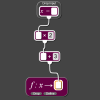### Exploring Simple Mappings

##### Age 11 to 14Challenge Level

Explore the relationship between simple linear functions and their graphs.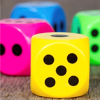### Dicey Operations

##### Age 11 to 14Challenge Level

Who said that adding, subtracting, multiplying and dividing couldn't be fun?### Nice or Nasty

##### Age 7 to 14Challenge Level

There are nasty versions of this dice game but we'll start with the nice ones...##### Age 7 to 14Challenge Level

Watch our videos of multiplication methods that you may not have met before. Can you make sense of them?### Diagonal Sums

##### Age 7 to 14Challenge Level

In this 100 square, look at the green square which contains the numbers 2, 3, 12 and 13. What is the sum of the numbers that are diagonally opposite each other? What do you notice?### How Many Miles to Go?

##### Age 11 to 14Challenge Level

How many more miles must the car travel before the numbers on the milometer and the trip meter contain the same digits in the same order?### What an Odd Fact(or)

##### Age 11 to 14Challenge Level

Can you show that 1^99 + 2^99 + 3^99 + 4^99 + 5^99 is divisible by 5?### Six Times Five

##### Age 11 to 14Challenge Level

How many six digit numbers are there which DO NOT contain a 5?### Think of Two Numbers

##### Age 11 to 14Challenge Level

Think of two whole numbers under 10, and follow the steps. I can work out both your numbers very quickly. How?### Number Rules - OK

##### Age 14 to 16Challenge Level

Can you produce convincing arguments that a selection of statements about numbers are true?### Two and Two

##### Age 11 to 16Challenge Level

How many solutions can you find to this sum? Each of the different letters stands for a different number.### Plus Minus

##### Age 14 to 16Challenge Level

Can you explain the surprising results Jo found when she calculated the difference between square numbers?### Legs Eleven

##### Age 11 to 14Challenge Level

Take any four digit number. Move the first digit to the end and move the rest along. Now add your two numbers. Did you get a multiple of 11?### Big Powers

##### Age 11 to 16Challenge Level

Three people chose this as a favourite problem. It is the sort of problem that needs thinking time - but once the connection is made it gives access to many similar ideas.### 2-digit Square

##### Age 14 to 16Challenge Level

A 2-Digit number is squared. When this 2-digit number is reversed and squared, the difference between the squares is also a square. What is the 2-digit number?### The Number Jumbler

##### Age 7 to 14Challenge Level

The Number Jumbler can always work out your chosen symbol. Can you work out how?### Reversals

##### Age 11 to 14Challenge Level

Where should you start, if you want to finish back where you started?##### Age 11 to 14Challenge Level

What happens when you add a three digit number to its reverse?##### Age 11 to 14Challenge Level

By selecting digits for an addition grid, what targets can you make?### Nice or Nasty for Two

##### Age 7 to 14Challenge Level

Some Games That May Be Nice or Nasty for an adult and child. Use your knowledge of place value to beat your opponent.### Always a Multiple?

##### Age 11 to 14Challenge Level

Think of a two digit number, reverse the digits, and add the numbers together. Something special happens...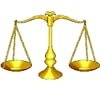### Balance Power

##### Age 11 to 18Challenge Level

Using balancing scales what is the least number of weights needed to weigh all integer masses from 1 to 1000? Placing some of the weights in the same pan as the object how many are needed?### A Story about Absolutely Nothing

##### Age 7 to 18

This article for the young and old talks about the origins of our number system and the important role zero has to play in it.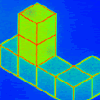### Pupils' Recording or Pupils Recording

##### Age 5 to 14

This article, written for teachers, looks at the different kinds of recordings encountered in Primary Mathematics lessons and the importance of not jumping to conclusions!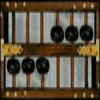##### Age 5 to 16

Nowadays the calculator is very familiar to many of us. What did people do to save time working out more difficult problems before the calculator existed?### Palindromes

##### Age 5 to 14### What a Joke

##### Age 14 to 16Challenge Level

Each letter represents a different positive digit AHHAAH / JOKE = HA What are the values of each of the letters?### Multiplication Magic

##### Age 14 to 16Challenge Level

Given any 3 digit number you can use the given digits and name another number which is divisible by 37 (e.g. given 628 you say 628371 is divisible by 37 because you know that 6+3 = 2+7 = 8+1 = 9). . . .### Composite Notions

##### Age 14 to 16Challenge Level

A composite number is one that is neither prime nor 1. Show that 10201 is composite in any base.### Arrange the Digits

##### Age 11 to 14Challenge Level

Can you arrange the digits 1,2,3,4,5,6,7,8,9 into three 3-digit numbers such that their total is close to 1500?### Phew I'm Factored

##### Age 14 to 16Challenge Level

Explore the factors of the numbers which are written as 10101 in different number bases. Prove that the numbers 10201, 11011 and 10101 are composite in any base.### Three Times Seven

##### Age 11 to 14Challenge Level

A three digit number abc is always divisible by 7 when 2a+3b+c is divisible by 7. Why?### Just Repeat

##### Age 11 to 14Challenge Level

Think of any three-digit number. Repeat the digits. The 6-digit number that you end up with is divisible by 91. Is this a coincidence?##### Age 11 to 18

We are used to writing numbers in base ten, using 0, 1, 2, 3, 4, 5, 6, 7, 8, and 9. Eg. 75 means 7 tens and five units. This article explains how numbers can be written in any number base.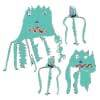### Back to the Planet of Vuvv

##### Age 11 to 14Challenge Level

There are two forms of counting on Vuvv - Zios count in base 3 and Zepts count in base 7. One day four of these creatures, two Zios and two Zepts, sat on the summit of a hill to count the legs of. . . .### More Mathematical Mysteries

##### Age 11 to 14Challenge Level

Write down a three-digit number Change the order of the digits to get a different number Find the difference between the two three digit numbers Follow the rest of the instructions then try. . . .### Eleven

##### Age 11 to 14Challenge Level

Replace each letter with a digit to make this addition correct.##### Age 11 to 14Challenge Level

Visitors to Earth from the distant planet of Zub-Zorna were amazed when they found out that when the digits in this multiplication were reversed, the answer was the same! Find a way to explain. . . .##### Age 11 to 14Challenge Level

Replace the letters with numbers to make the addition work out correctly. R E A D + T H I S = P A G E### Back to Basics

##### Age 14 to 16Challenge Level

Find b where 3723(base 10) = 123(base b).### X Marks the Spot

##### Age 11 to 14Challenge Level

When the number x 1 x x x is multiplied by 417 this gives the answer 9 x x x 0 5 7. Find the missing digits, each of which is represented by an "x" .### Really Mr. Bond

##### Age 14 to 16Challenge Level

115^2 = (110 x 120) + 25, that is 13225 895^2 = (890 x 900) + 25, that is 801025 Can you explain what is happening and generalise?### Enriching Experience

##### Age 14 to 16Challenge Level

Find the five distinct digits N, R, I, C and H in the following nomogram### Basically

##### Age 11 to 14Challenge Level

The number 3723(in base 10) is written as 123 in another base. What is that base?### Tis Unique

##### Age 11 to 14Challenge Level

This addition sum uses all ten digits 0, 1, 2...9 exactly once. Find the sum and show that the one you give is the only possibility.### Chocolate Maths

##### Age 11 to 14Challenge Level

Pick the number of times a week that you eat chocolate. This number must be more than one but less than ten. Multiply this number by 2. Add 5 (for Sunday). Multiply by 50... Can you explain why it. . . .### Cayley

##### Age 11 to 14Challenge Level

The letters in the following addition sum represent the digits 1 ... 9. If A=3 and D=2, what number is represented by "CAYLEY"?### Quick Times

##### Age 11 to 14Challenge Level

32 x 38 = 30 x 40 + 2 x 8; 34 x 36 = 30 x 40 + 4 x 6; 56 x 54 = 50 x 60 + 6 x 4; 73 x 77 = 70 x 80 + 3 x 7 Verify and generalise if possible.### Never Prime

##### Age 14 to 16Challenge Level

If a two digit number has its digits reversed and the smaller of the two numbers is subtracted from the larger, prove the difference can never be prime.